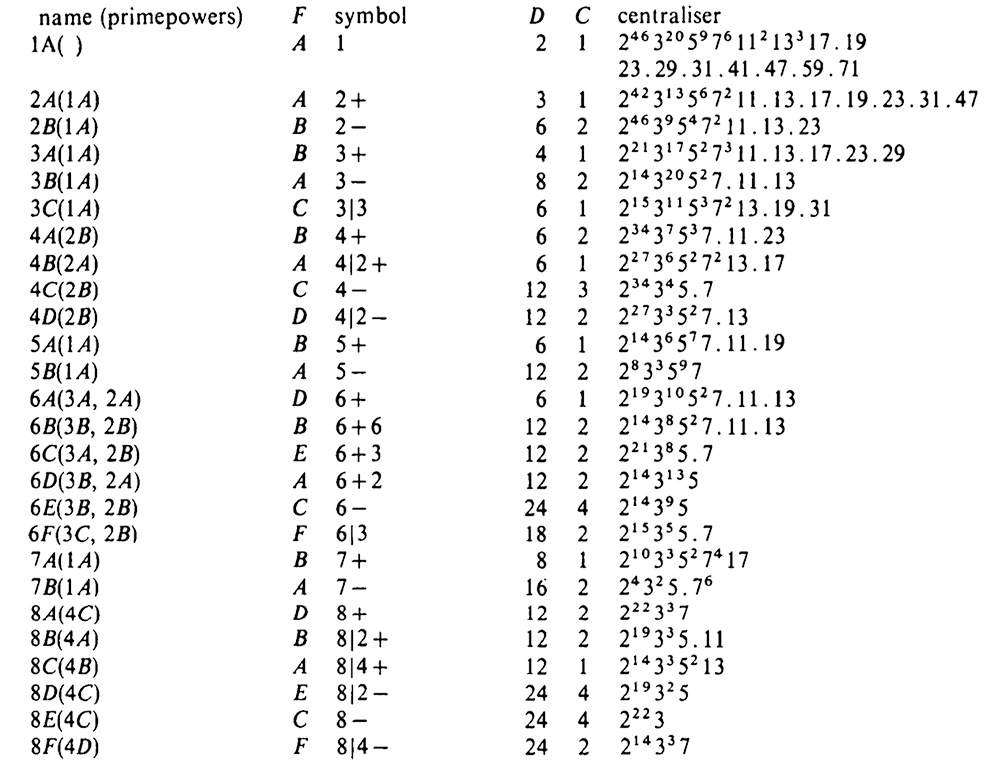# Tag: McKay

Whenever I visit someone’s YouTube or Twitter profile page, I hope to see an interesting banner image. Here’s the one from Richard Borcherds’ YouTube Channel.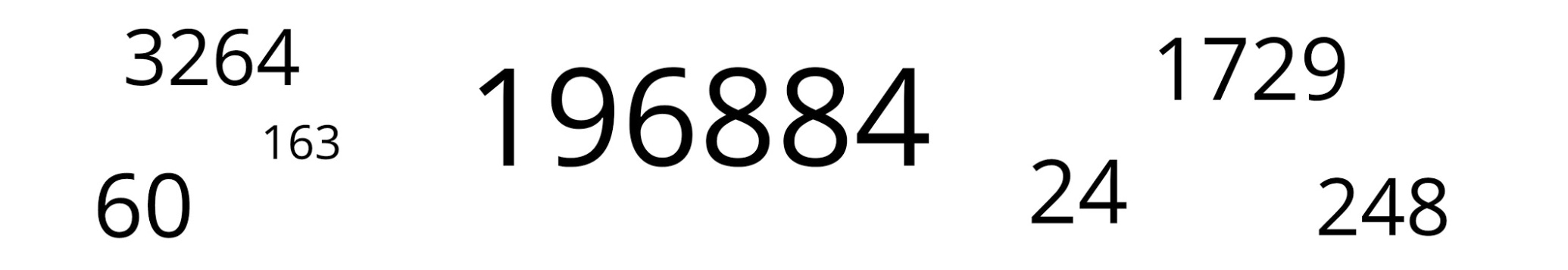Not too surprisingly for Borcherds, almost all of these numbers are related to the monster group or its moonshine.

Let’s try to decode them, in no particular order.

196884

John McKay’s observation $196884 = 1 + 196883$ was the start of the whole ‘monstrous moonshine’ industry. Here, $1$ and $196883$ are the dimensions of the two smallest irreducible representations of the monster simple group, and $196884$ is the first non-trivial coefficient in Klein’s j-function in number theory.

$196884$ is also the dimension of the space in which Robert Griess constructed the Monster, following Simon Norton’s lead that there should be an algebra structure on the monster-representation of that dimension. This algebra is now known as the Griess algebra.

Here’s a recent talk by Griess “My life and times with the sporadic simple groups” in which he tells about his construction of the monster (relevant part starting at 1:15:53 into the movie).

1729

1729 is the second (and most famous) taxicab number. A long time ago I did write a post about the classic Ramanujan-Hardy story the taxicab curve (note to self: try to tidy up the layout of some old posts!).

Recently, connections between Ramanujan’s observation and K3-surfaces were discovered. Emory University has an enticing press release about this: Mathematicians find ‘magic key’ to drive Ramanujan’s taxi-cab number. The paper itself is here.

“We’ve found that Ramanujan actually discovered a K3 surface more than 30 years before others started studying K3 surfaces and they were even named. It turns out that Ramanujan’s work anticipated deep structures that have become fundamental objects in arithmetic geometry, number theory and physics.”

Ken Ono

24

There’s no other number like $24$ responsible for the existence of sporadic simple groups.

24 is the length of the binary Golay code, with isomorphism group the sporadic Mathieu group $M_24$ and hence all of the other Mathieu-groups as subgroups.

24 is the dimension of the Leech lattice, with isomorphism group the Conway group $Co_0 = .0$ (dotto), giving us modulo its center the sporadic group $Co_1=.1$ and the other Conway groups $Co_2=.2, Co_3=.3$, and all other sporadics of the second generation in the happy family as subquotients (McL,HS,Suz and $HJ=J_2$)24 is the central charge of the Monster vertex algebra constructed by Frenkel, Lepowski and Meurman. Most experts believe that the Monster’s reason of existence is that it is the symmetry group of this vertex algebra. John Conway was one among few others hoping for a nicer explanation, as he said in this interview with Alex Ryba.

24 is also an important number in monstrous moonshine, see for example the post the defining property of 24. There’s a lot more to say on this, but I’ll save it for another day.

60

60 is, of course, the order of the smallest non-Abelian simple group, $A_5$, the rotation symmetry group of the icosahedron. $A_5$ is the symmetry group of choice for most viruses but not the Corona-virus.

3264

3264 is the correct solution to Steiner’s conic problem asking for the number of conics in $\mathbb{P}^2_{\mathbb{C}}$ tangent to five given conics in general position.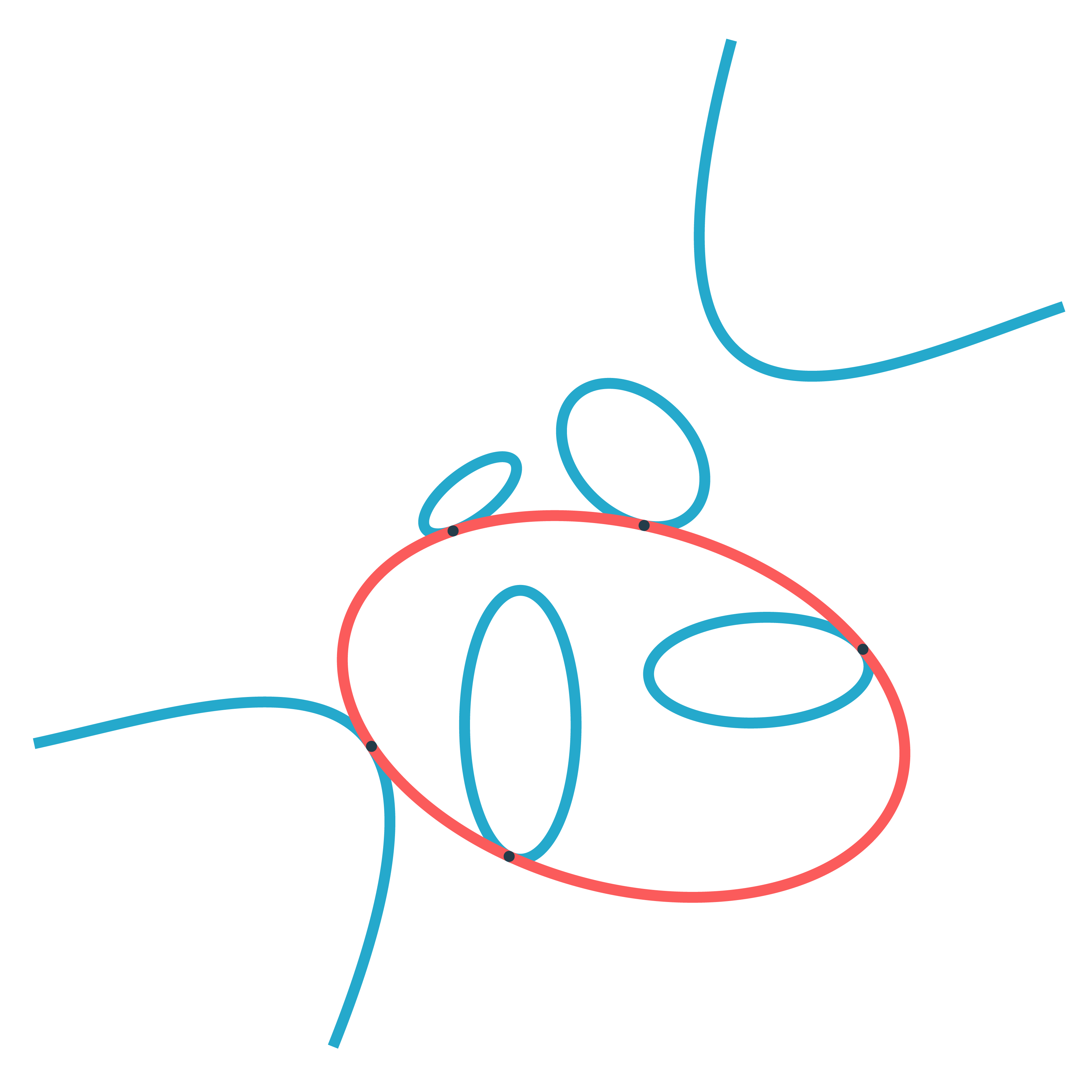Steiner himself claimed that there were $7776=6^5$ such conics, but realised later that he was wrong. The correct number was first given by Ernest de Jonquières in 1859, but a rigorous proof had to await the advent of modern intersection theory.

Eisenbud and Harris wrote a book on intersection theory in algebraic geometry, freely available online: 3264 and all that.

248

248 is the dimension of the exceptional simple Lie group $E_8$. $E_8$ is also connected to the monster group.

If you take two Fischer involutions in the monster (elements of conjugacy class 2A) and multiply them, the resulting element surprisingly belongs to one of just 9 conjugacy classes:

1A,2A,2B,3A,3C,4A,4B,5A or 6A

The orders of these elements are exactly the dimensions of the fundamental root for the extended $E_8$ Dynkin diagram.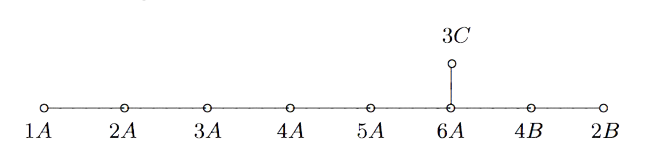This is yet another moonshine observation by John McKay and I wrote a couple of posts about it and about Duncan’s solution: the monster graph and McKay’s observation, and $E_8$ from moonshine groups.

163

163 is a remarkable number because of the ‘modular miracle’
$e^{\pi \sqrt{163}} = 262537412640768743.99999999999925…$
This is somewhat related to moonshine, or at least to Klein’s j-function, which by a result of Kronecker’s detects the classnumber of imaginary quadratic fields $\mathbb{Q}(\sqrt{-D})$ and produces integers if the classnumber is one (as is the case for $\mathbb{Q}(\sqrt{-163})$).

The details are in the post the miracle of 163, or in the paper by John Stillwell, Modular Miracles, The American Mathematical Monthly, 108 (2001) 70-76.

His description of the $j$-function (at 4:13 in the movie) is simply hilarious!

Borcherds connects $163$ to the monster moonshine via the $j$-function, but there’s another one.

The monster group has $194$ conjugacy classes and monstrous moonshine assigns a ‘moonshine function’ to each conjugacy class (the $j$-function is assigned to the identity element). However, these $194$ functions are not linearly independent and the space spanned by them has dimension exactly $163$.

Dedekind’s Psi-function $\Psi(n)= n \prod_{p |n}(1 + \frac{1}{p})$ pops up in a number of topics:

• $\Psi(n)$ is the index of the congruence subgroup $\Gamma_0(n)$ in the modular group $\Gamma=PSL_2(\mathbb{Z})$,
• $\Psi(n)$ is the number of points in the projective line $\mathbb{P}^1(\mathbb{Z}/n\mathbb{Z})$,
• $\Psi(n)$ is the number of classes of $2$-dimensional lattices $L_{M \frac{g}{h}}$ at hyperdistance $n$ in Conway’s big picture from the standard lattice $L_1$,
• $\Psi(n)$ is the number of admissible maximal commuting sets of operators in the Pauli group of a single qudit.

The first and third interpretation have obvious connections with Monstrous Moonshine.

Conway’s big picture originated from the desire to better understand the Moonshine groups, and Ogg’s Jack Daniels problem
asks for a conceptual interpretation of the fact that the prime numbers such that $\Gamma_0(p)^+$ is a genus zero group are exactly the prime divisors of the order of the Monster simple group.

Here’s a nice talk by Ken Ono : Can’t you just feel the Moonshine?

For this reason it might be worthwhile to make the connection between these two concepts and the number of points of $\mathbb{P}^1(\mathbb{Z}/n\mathbb{Z})$ as explicit as possible.

Surely all of this is classical, but it is nicely summarised in the paper by Tatitscheff, He and McKay “Cusps, congruence groups and monstrous dessins”.

The ‘monstrous dessins’ from their title refers to the fact that the lattices $L_{M \frac{g}{h}}$ at hyperdistance $n$ from $L_1$ are permuted by the action of the modular groups and so determine a Grothendieck’s dessin d’enfant. In this paper they describe the dessins corresponding to the $15$ genus zero congruence subgroups $\Gamma_0(n)$, that is when $n=1,2,3,4,5,6,7,8,9,10,12,13,16,18$ or $25$.

Here’s the ‘monstrous dessin’ for $\Gamma_0(6)$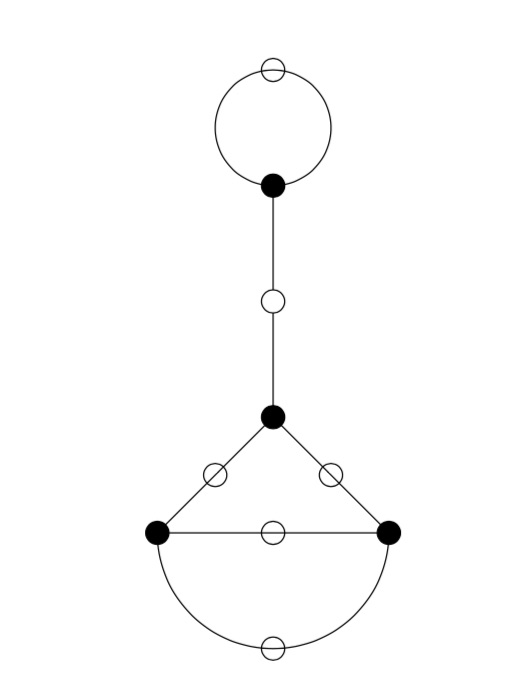But, one can compute these dessins for arbitrary $n$, describing the ripples in Conway’s big picture, and try to figure out whether they are consistent with the Riemann hypothesis.

We will get there eventually, but let’s start at an easy pace and try to describe the points of the projective line $\mathbb{P}^1(\mathbb{Z}/n \mathbb{Z})$.

Over a field $k$ the points of $\mathbb{P}^1(k)$ correspond to the lines through the origin in the affine plane $\mathbb{A}^2(k)$ and they can represented by projective coordinates $[a:b]$ which are equivalence classes of couples $(a,b) \in k^2- \{ (0,0) \}$ under scalar multiplication with non-zero elements in $k$, so with points $[a:1]$ for all $a \in k$ together with the point at infinity $[1:0]$. When $n=p$ is a prime number we have $\# \mathbb{P}^1(\mathbb{Z}/p\mathbb{Z}) = p+1$. Here are the $8$ lines through the origin in $\mathbb{A}^2(\mathbb{Z}/7\mathbb{Z})$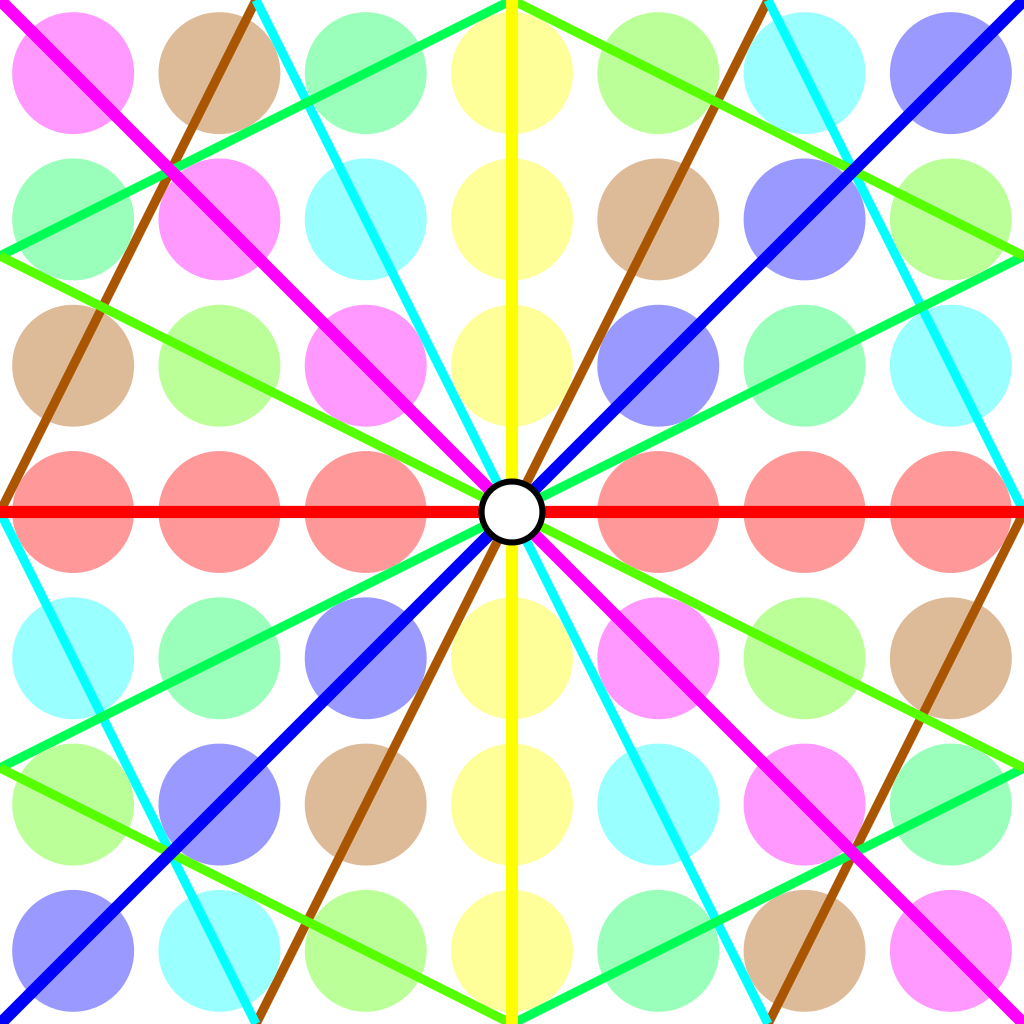Over an arbitrary (commutative) ring $R$ the points of $\mathbb{P}^1(R)$ again represent equivalence classes, this time of pairs
$(a,b) \in R^2~:~aR+bR=R$
with respect to scalar multiplication by units in $R$, that is
$(a,b) \sim (c,d)~\quad~\text{iff}~\qquad \exists \lambda \in R^*~:~a=\lambda c, b = \lambda d$
For $\mathbb{P}^1(\mathbb{Z}/n \mathbb{Z})$ we have to find all pairs of integers $(a,b) \in \mathbb{Z}^2$ with $0 \leq a,b < n$ with $gcd(a,b)=1$ and use Cremona’s trick to test for equivalence:
$(a,b) = (c,d) \in \mathbb{P}^1(\mathbb{Z}/n \mathbb{Z})~\quad \text{iff}~\quad ad-bc \equiv 0~mod~n$
The problem is to find a canonical representative in each class in an efficient way because this is used a huge number of times in working with modular symbols.

Perhaps the best algorithm, for large $n$, is sketched in pages 145-146 of Bill Stein’s Modular forms: a computational approach.

For small $n$ the algorithm in $\S 1.3$ in the Tatitscheff, He and McKay paper suffices:

• Consider the action of $(\mathbb{Z}/n\mathbb{Z})^*$ on $\{ 0,1,…,n-1 \}=\mathbb{Z}/n\mathbb{Z}$ and let $D$ be the set of the smallest elements in each orbit,
• For each $d \in D$ compute the stabilizer subgroup $G_d$ for this action and let $C_d$ be the set of smallest elements in each $G_d$-orbit on the set of all elements in $\mathbb{Z}/n \mathbb{Z}$ coprime with $d$,
• Then $\mathbb{P}^1(\mathbb{Z}/n\mathbb{Z})= \{ [c:d]~|~d \in D, c \in C_d \}$.

Let’s work this out for $n=12$ which will be our running example (the smallest non-squarefree non-primepower):

• $(\mathbb{Z}/12\mathbb{Z})^* = \{ 1,5,7,11 \} \simeq C_2 \times C_2$,
• The orbits on $\{ 0,1,…,11 \}$ are
$\{ 0 \}, \{ 1,5,7,11 \}, \{ 2,10 \}, \{ 3,9 \}, \{ 4,8 \}, \{ 6 \}$
and $D=\{ 0,1,2,3,4,6 \}$,
• $G_0 = C_2 \times C_2$, $G_1 = \{ 1 \}$, $G_2 = \{ 1,7 \}$, $G_3 = \{ 1,5 \}$, $G_4=\{ 1,7 \}$ and $G_6=C_2 \times C_2$,
• $1$ is the only number coprime with $0$, giving us $[1:0]$,
• $\{ 0,1,…,11 \}$ are all coprime with $1$, and we have trivial stabilizer, giving us the points $[0:1],[1:1],…,[11:1]$,
• $\{ 1,3,5,7,9,11 \}$ are coprime with $2$ and under the action of $\{ 1,7 \}$ they split into the orbits
$\{ 1,7 \},~\{ 3,9 \},~\{ 5,11 \}$
giving us the points $[1:2],[3:2]$ and $[5:2]$,
• $\{ 1,2,4,5,7,8,10,11 \}$ are coprime with $3$, the action of $\{ 1,5 \}$ gives us the orbits
$\{ 1,5 \},~\{ 2,10 \},~\{ 4,8 \},~\{ 7,11 \}$
and additional points $[1:3],[2:3],[4:3]$ and $[7:3]$,
• $\{ 1,3,5,7,9,11 \}$ are coprime with $4$ and under the action of $\{ 1,7 \}$ we get orbits
$\{ 1,7 \},~\{ 3,9 \},~\{ 5,11 \}$
and points $[1:4],[3:4]$ and $[5,4]$,
• Finally, $\{ 1,5,7,11 \}$ are the only coprimes with $6$ and they form a single orbit under $C_2 \times C_2$ giving us just one additional point $[1:6]$.

This gives us all $24= \Psi(12)$ points of $\mathbb{P}^1(\mathbb{Z}/12 \mathbb{Z})$ (strangely, op page 43 of the T-H-M paper they use different representants).

One way to see that $\# \mathbb{P}^1(\mathbb{Z}/n \mathbb{Z}) = \Psi(n)$ comes from a consequence of the Chinese Remainder Theorem that for the prime factorization $n = p_1^{e_1} … p_k^{e_k}$ we have
$\mathbb{P}^1(\mathbb{Z}/n \mathbb{Z}) = \mathbb{P}^1(\mathbb{Z}/p_1^{e_1} \mathbb{Z}) \times … \times \mathbb{P}^1(\mathbb{Z}/p_k^{e_k} \mathbb{Z})$
and for a prime power $p^k$ we have canonical representants for $\mathbb{P}^1(\mathbb{Z}/p^k \mathbb{Z})$
$[a:1]~\text{for}~a=0,1,…,p^k-1~\quad \text{and} \quad [1:b]~\text{for}~b=0,p,2p,3p,…,p^k-p$
which shows that $\# \mathbb{P}^1(\mathbb{Z}/p^k \mathbb{Z}) = (p+1)p^{k-1}= \Psi(p^k)$.

Next time, we’ll connect $\mathbb{P}^1(\mathbb{Z}/n \mathbb{Z})$ to Conway’s big picture and the congruence subgroup $\Gamma_0(n)$.

If you take two Fischer involutions in the monster (elements of conjugacy class 2A) and multiply them, the resulting element surprisingly belongs to one of just 9 conjugacy classes:

1A,2A,2B,3A,3C,4A,4B,5A or 6A

The orders of these elements are exactly the dimensions of the fundamental root for the extended $E_8$ Dynkin diagram.

This is the content of John McKay’s E(8)-observation : there should be a precise relation between the nodes of the extended Dynkin diagram and these 9 conjugacy classes in such a way that the order of the class corresponds to the component of the fundamental root. More precisely, one conjectures the following correspondence:John Duncan found such a connection by considering carefully the corresponding moonshine groups and their inter-relation. For more on this, look at the old post E8 from moonshine groups. The extended Dynkin diagram with these moonshine groups as vertices is: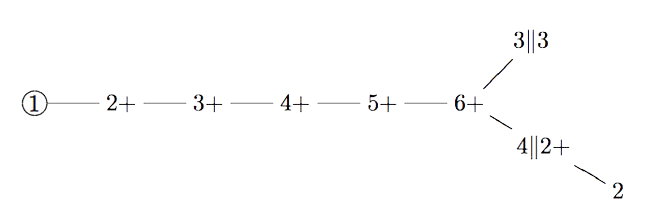Duncan does this by assigning numbers to moonshine groups: the dimension is the order of the corresponding monster element and the valency is one more than the copies of $C_2$ generated by the Atkin-Lehner involutions in the moonshine group.

One might ask whether there is a graph on all 171 moonshine groups, compatible with the valencies of every vertex.

Now, even for the 9 groups in McKay’s question, the valencies do not determine the graph uniquely and Duncan proceeds with an ad hoc condition on the edges.

There is a partition on the 9 groups by the property whether or not the index of the intersection with $\Gamma_0(2)$ is at most two. Then Duncan declares that there cannot be an edge between two groups belonging to the same class.

His motivation for this property comes from classical McKay-correspondence for the binary icosahedral group (where the vertices correspond to simple representations $S$, and the edges from $S$ to factors of $S \otimes V_2$, where $V_2$ is the restriction of the standard $2$-dimensional simple for $SU(2)$).

Of the $9$ simples there are only $4$ faithful ones, $5$ come from simples of $A_5$. Because $\Gamma_0(2)$ is a subgroup of the modular group of index 2, he then views $\Gamma_0(2)$ as similar to the subgroup $A_5$ in the binary icosahedral group, and declares a moonshine group to be faithful if its index in the intersection with $\Gamma_0(2)$ is at most two.

One might ask whether there is another, more natural, definition for having an edge (or multiple ones) between arbitrary moonshine groups.

And, what is the full graph on the 171 groups?

One of the better opening quotes of a paper:

“Even quite ungainly objects, like chairs and tables, will become almost spherical if you wrap them in enough newspaper.”The paper in question is The orbifold notation for surface groups by John Conway.

Here’s Conway talking leisurely about Thurston’s idea to capture the acting group via the topology of the orbifold space and his own notation for such orbifolds.

Here’s another version of the paper, with illustrations: The orbifold notation for two-dimensional groups, by Conway and Daniel H. Huson.

A very accessible account are these lecture notes:

A field guide to the orbifolds, notes from class on “Geometry and the Imagination” in Minneapolis, with John Conway, Peter Doyle, Jane Gilman and Bill Thurston, on June 17–28, 1991.

And, here are notes by Thurston on The Geometry and Topology of Three-Manifolds, including stuff about orbifolds.

I came across these papers struggling my way through On the discrete groups of moonshine by Conway, McKay and Sebbar.

On the genus $0$ property of moonshine groups they have this to say:

“As for groups of the form $(n|h)+e,f,\dots$, the genus can be determined from the fundamental regions using the Riemann-Hurwitz formula. Since most of the groups are not subgroups of the modular group, the calculations of the genus, which cannot be produced here because of their length, are carried out by finding the elliptic fixed points and the cone points in the orbifolds attached to the fundamental regions. The Euler characteristic of the orbifold determines the genus of the group. See [paper] for more details on orbifold techniques.”

Monstrous moonshine associates to every element of order $n$ of the monster group $\mathbb{M}$ an arithmetic group of the form
$(n|h)+e,f,\dots$
where $h$ is a divisor of $24$ and of $n$ and where $e,f,\dots$ are divisors of $\frac{n}{h}$ coprime with its quotient.

In snakes, spines, and all that we’ve constructed the arithmetic group
$\Gamma_0(n|h)+e,f,\dots$
which normalizes $\Gamma_0(N)$ for $N=h.n$. If $h=1$ then this group is the moonshine group $(n|h)+e,f,\dots$, but for $h > 1$ the moonshine group is a specific subgroup of index $h$ in $\Gamma_0(n|h)+e,f,\dots$.

I’m sure one can describe this subgroup explicitly in each case by analysing the action of the finite group $(\Gamma_0(n|h)+e,f,\dots)/\Gamma_0(N)$ on the $(N|1)$-snake. Some examples were worked out by John Duncan in his paper Arithmetic groups and the affine E8 Dynkin diagram.

But at the moment I don’t understand the general construction given by Conway, McKay and Sebbar in On the discrete groups of moonshine. I’m stuck at the last sentence of (2) in section 3. Nothing a copy of Charles Ferenbaugh Ph. D. thesis cannot fix.

The correspondence between the conjugacy classes of the Monster and these arithmetic groups takes up 3 pages in Conway & Norton’s Monstrous Moonshine. Here’s the beginning of it.